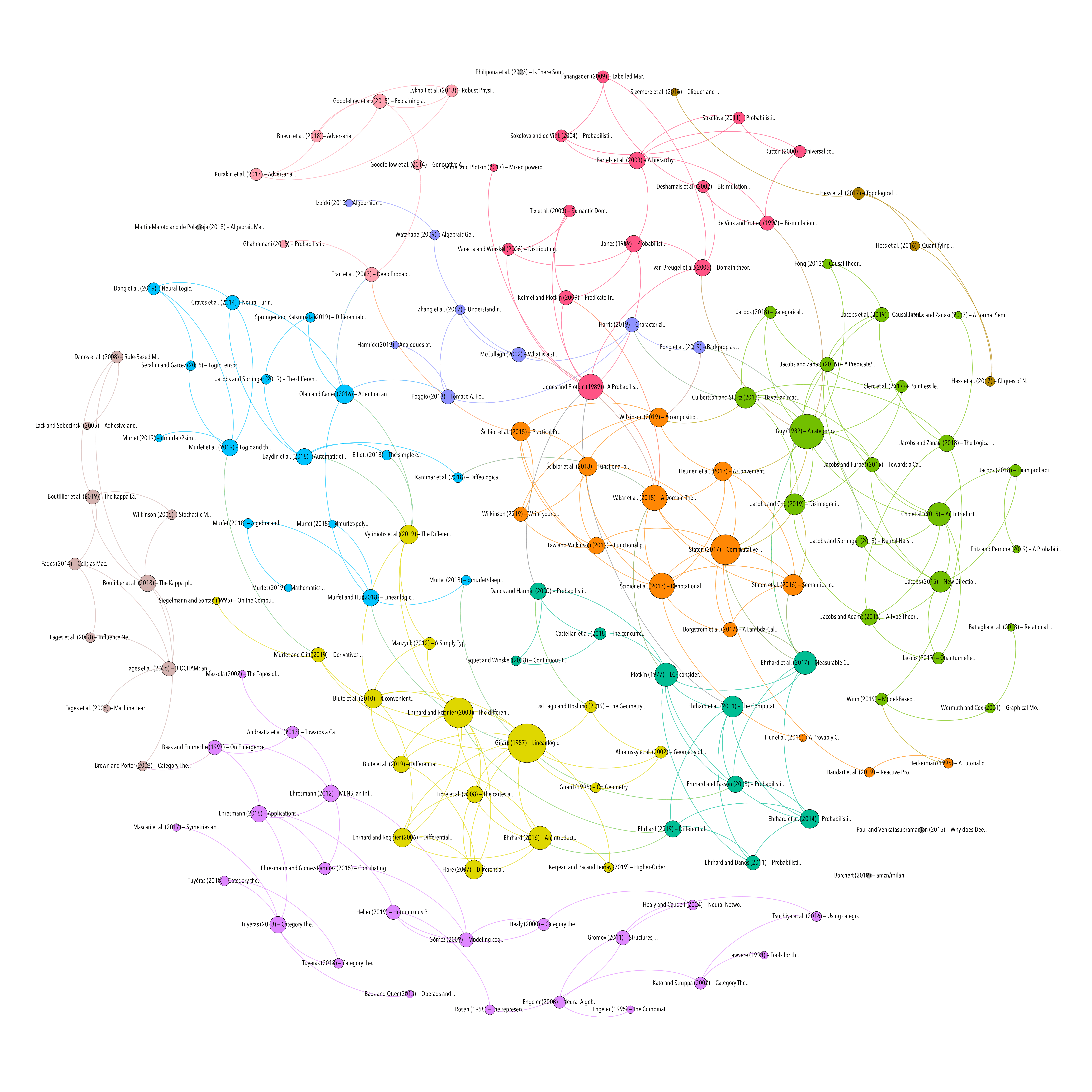# Full bibliography

## Functional probabilistic programming for scalable Bayesian modelling

Resource type
Authors/contributors
Title
Functional probabilistic programming for scalable Bayesian modelling
Abstract
Bayesian inference involves the specification of a statistical model by a statistician or practitioner, with careful thought about what each parameter represents. This results in particularly interpretable models which can be used to explain relationships present in the observed data. Bayesian models are useful when an experiment has only a small number of observations and in applications where transparency of data driven decisions is important. Traditionally, parameter inference in Bayesian statistics has involved constructing bespoke MCMC (Markov chain Monte Carlo) schemes for each newly proposed statistical model. This results in plausible models not being considered since efficient inference schemes are challenging to develop or implement. Probabilistic programming aims to reduce the barrier to performing Bayesian inference by developing a domain specific language (DSL) for model specification which is decoupled from the parameter inference algorithms. This paper introduces functional programming principles which can be used to develop an embedded probabilistic programming language. Model inference can be carried out using any generic inference algorithm. In this paper Hamiltonian Monte Carlo (HMC) is used, an efficient MCMC method requiring the gradient of the un-normalised log-posterior, calculated using automatic differentiation. The concepts are illustrated using the Scala programming language.
Publication
arXiv:1908.02062 [stat]
Date
2019-08-06
Accessed
2019-11-27T20:48:16Z
Library Catalog
Extra
ZSCC: 0000000 arXiv: 1908.02062
Citation
Law, J., & Wilkinson, D. (2019). Functional probabilistic programming for scalable Bayesian modelling. ArXiv:1908.02062 [Stat]. Retrieved from http://arxiv.org/abs/1908.02062
DIFFERENTIAL CALCULUS
PROBABILITY & STATISTICS
PROGRAMMING LANGUAGES
Methodology
Topic
Attachment
Processing time: 0.02 secondsGraph of references (from Zotero to Gephi via Zotnet with this script)